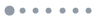# 小ben成长手册 欢迎光临

• 本站统计
• 文章总数:583篇
• 评论总数:966条
• 分类总数:43个
• 标签总数:459个
• 友链总数:66个
• 建站日期:2016-05-11
• Statics2
作者: other | 发布时间: 2020-11-01 20:26:11 | 点击量: 754
标签:

1.3 Measurement for average level

Numerical characteristics:

Average level

Variation

1.3.1 Arithmetic mean

Useful when the histogram looks symmetric.

Denote the observed values of the individuals

with x1, x2,... xn, the arithmetic mean

x= (x1+x2+...xn) /n

1.3.2 Geometric mean

it is useful when the histogram of the logarithms is

close to symmetric.

1.3.3 Median

when the histogram shows skew, the median can

be applied to measure the average level

median = the value in the middle

when n is odd,

when n is even,

25 percentile P25:

75 percentile P75:

P2.5

P97.5

名称(*)

邮箱

网址

二十二加三等于几？

(*)

本站资源均来自网络，如有侵权请联系我们删除e-mail:benen005@sina.com